# 基于有向赋权图的RGV动态调度策略研究Dynamic Schedule Strategy of RGV Based on the Weighted Directed Graph

• 全文下载: PDF(1047KB)    PP.195-203   DOI: 10.12677/PM.2019.92025
• 下载量: 314  浏览量: 650

To solve the RGV scheduling problem, a RGV dynamic scheduling model based on graph theory is established. We transform distance into time in the problem so that the practical problem can be transformed into a mathematical model represented by a weighted directed graph, and virtual nodes are set up to solve the situation that the time is zero. In the one process, we schedule dy-namically based on Greedy Algorithm. In each step, Floyd algorithm is used to search the virtual node with the smallest total distance from current of RGV position weight to determine the next moving node of RGV. In the two processes, we improve the traditional Simulated Annealing Algo-rithm (SA) based on Particle Swarm Optimization (PSO), and combine the scheduling algorithm in the one process. Aiming at maximizing the output, the optimal allocation of CNCs is provided, and then the optimal scheduling strategy of RGV is obtained within the specified working time.

1. 引言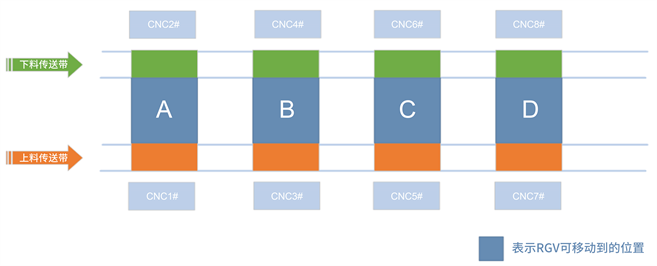Figure 1. Schematic diagram of RGV working principle

2. 模型假设与符号说明(表1)

1) 假设上下料传送带都是连续不断的，能及时为RGV提供生料和运输熟料；

2) 假设RGV在完成上下料作业后即刻进入清洗作业阶段；

3) 假设每班次连续工作八小时；

4) 作业开始时，RGV的初始位置位于1号CNC前；

5) 假设该班次结束时仍处于加工状态的物料不计入生产件数。

3. RGV动态调度问题的数学模型

3.1. 节点说明

3.2. 权值说明

$<{v}_{i},{v}_{j}>$ 表示从 ${v}_{i}$${v}_{j}$ 的一条边， ${t}_{ij}$ 为对应权值，同时也表示相应的工作时间或移动时间，其中 $i$ ， 表示任一节点。图的边集E包含以下三个部分：

1) RGV位置节点 ${v}_{A},{v}_{B},{v}_{C},{v}_{D}$ 之间的权值。

2) RGV位置节点(用 $i$ 表示)与CNC位置节点(用j表示)间的权值。弧 $〈{v}_{i},{v}_{j}〉$ 表示RGV为CNC上下料，权值为上下料所需时间。弧 $〈{v}_{i},{v}_{j}〉$ 表示RGV清洗熟料，当CNC未加工处于空闲时，无熟料产生，下料为空，不进行清洗操作，权值为0 s；当CNC已加工完处于空闲时，有熟料产生，进入清洗作业阶段，权值为清洗作业所需时间 ${t}_{q}$ ，如公式(1)所示。

${t}_{ij}=\left\{\begin{array}{cc}0& \text{CNC}未加工空闲\\ {t}_{q}& \text{CNC}加工完毕空闲\end{array}$ (1)

3) 环 $<{v}_{i},{v}_{j}>$ 表示RGV不进行任何操作，权值为等待时间。因此，图 $\left(V,E\right)$ 的点集和边集  可表示为：

$V=\left\{{v}_{i}|i=A,\cdots ,D,1,\cdots ,8,{1}^{\prime },\cdots ,{8}^{\prime }\right\},E=\left\{〈{v}_{i},{v}_{j}〉|i,j=A,\cdots ,D,1,\cdots ,8,{1}^{\prime },\cdots ,{8}^{\prime }\right\}$ (2)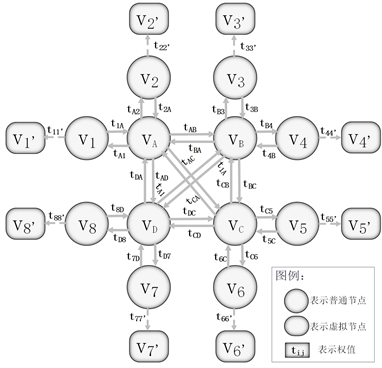Figure 2. Scheduling optimization of network diagram

RGV作业周期  ：当工作状态达到稳定后，RGV访问CNC的次序不再改变，将此时依次访问各CNC编号序列的最小重复单元个数 $n$ 定义为周期长度，则RGV 连续访问 $n$ 个CNC可定义为一个作业周期。

RGV作业序列：将一个周期内RGV依次访问各CNC的编号顺序定义为作业序列，并表示为：

$Sep=\left({\lambda }_{1}\to {\lambda }_{2}\to \cdots \to {\lambda }_{n}\to {\lambda }_{1}\right)$ (3)

4. 基于图论的RGV动态调度算法

4.1. 一道工序物料加工作业——算法一：基于贪心算法的RGV动态调度算法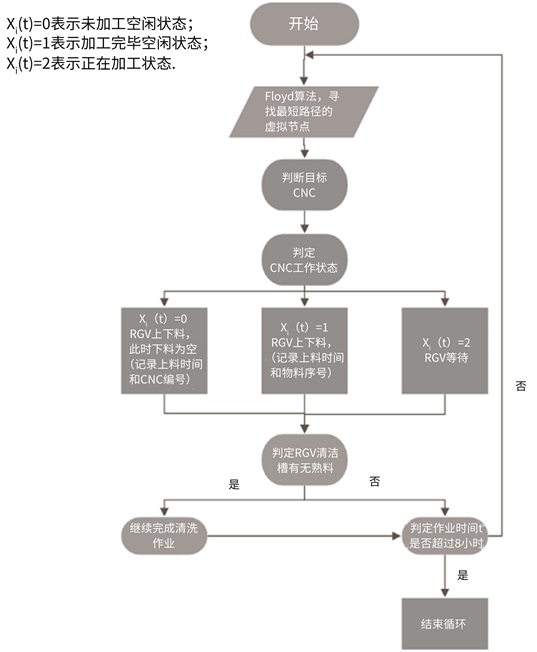Figure 3. Solution flow based on Greedy Algorithm

${X}_{i}\left(t\right)$ 表示CNC工作状态：

${X}_{i}\left(t\right)=\left\{\begin{array}{cc}0& \text{CNC}未加工空闲状态\\ 1& \text{CNC}加工完毕空闲状态\\ 2& \text{CNC}加工完毕空闲状态\end{array}$ (4)

$RGVF\left(t\right)$ 表示RGV清洁槽内是否有熟料：

$RGVF\left(t\right)=\left\{\begin{array}{cc}0& 无熟料\\ 1& 有熟料\end{array}$ (5)

1) 当 ${X}_{i}\left(t\right)$ = 0时，CNC处于空闲状态，等待0 s，RGV进行上下料，但下料为空并记录上料时间和CNC编号；

2) 当 ${X}_{i}\left(t\right)$ = 1时，CNC已加工完毕，等待0 s，RGV进行上下料，记录上下料时间和物料序号 ${Z}_{i}\left(t\right)$

3) 当 ${X}_{i}\left(t\right)$ = 2时，CNC正在加工，等待剩余加工时间为 ${Y}_{i}\left(t\right)$

4.2. 两道工序物料加工作业——算法二：改进型模拟退火算法

4.2.1. 算法一的改进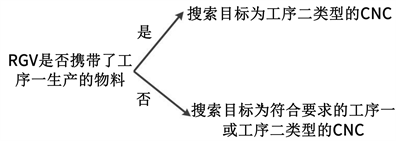4.2.2. 改进模拟退火算法

1) 算法二迭代过程

a) 生成m个粒子作为初始种群，赋给粒子的位置初值，给定初始接受概率p、初始温度T、终止温度 ${T}_{e}$ 、温度动态衰减系数 $k$ 、迭代次数 $n$

b) 判断算法是否达到循环终止条件：

i) 循环次数 $r$ 从1循环至 $n$ ；ii) 体系降温至终止温度的 ${T}_{e}$

c) 计算每个粒子适应度 ${f}_{i}\left(r\right)$${f}_{\text{agv}}\left(r\right)$

i) 若粒子的适应度优于原个体极值 ${p}_{\text{best}}$ ，则进行更新并选择最优个体值作为群体极值 ${g}_{\text{best}}$

ii) 若粒子的适应度不能优于原个体极值 ${p}_{\text{best}}$ ，则更新 ${p}_{0}=\mathrm{exp}\left({-1/p\cdot T}_{0}\right)$ ，并以 ${p}_{0}$ 为概率接受当前适应度作为 ${p}_{\text{best}}$ ；若未接受粒子的新适应度，则不做更新。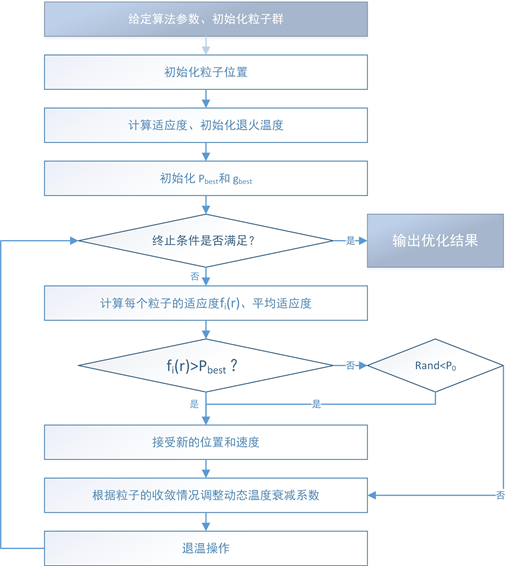Figure 4. Improved simulated annealing algorithm

d) 在每个粒子当前位置中增加一个服从正态分布的随机值作为新的粒子位置。

e) 根据粒子个体适应度和平均适应度，计算温度自动衰减系数k。

f) 更新当前环境温度： ${T}_{0}=k\cdot {T}_{e}$

2) 退火速度的改进

$K=\mu +\left[1-\mathrm{exp}\left({f}_{\text{pi}}-{f}_{\text{avg}}\right)\right]\cdot N\left(0,1\right)/\left(2{T}_{r-1}\right)$ (6)

3) 最优分配的计算

a) 生成服从均匀分布 $U\left(0,256\right)$ 的随机数并取整数后得到粒子初始位置，并转为八位二进制编码(不足八位在数字最前补零)。加工第一道工序的CNC用“1”表示，加工第二道工序的CNC用“0”表示，第 位数字表示编号为 $i$ 的CNC。

b) 将得到的二进制八位数代入基于贪心算法的求解算法中得到当前粒子位置对应的适应度 ${f}_{i}$ ，即八小时的生产件数。

c) 带入算法中迭代求得函数最优值 ${g}_{\text{best}}$ ，并将其对应的粒子位置转化为八位二进制数字，即得机器工序的最优分配。

5. 仿真结果分析Table 2. System operating parametersTable 3. Production situation of material processing in single procedureTable 4. Production situation of material processing in double procedure

6. 结论

  中国工业与应用数学协会. 全国大学生数学建模竞赛[Z]. B题2018.  严蔚敏. 数据结构[M]. 北京: 清华大学出版社, 1992.  潘全科, 朱剑英. 作业车间动态调度研究[J]. 南京航空航天大学学报, 2005, 37(2): 262-268.  汪莹. 论贪心算法在图论中的应用[J]. 计算机光盘软件与应用, 2013(16): 309 + 311.  刘爱军, 杨育, 李斐, 邢青松, 陆惠, 张煜东. 混沌模拟退火粒子群优化算法研究及应用[J]. 浙江大学学报(工学版), 2013, 47(10): 1722-1730.